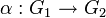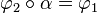# Restriction functor on cohomology

## Definition

### General definition

Suppose$G_1,G_2$ are groups and$A$ is an abelian group. Suppose$\varphi_1:G_1 \to \operatorname{Aut}(A)$,$\varphi_2: G_2 \to \operatorname{Aut}(A)$, and$\alpha: G_1 \to G_2$ are homomorphisms such that$\varphi_2 \circ \alpha = \varphi_1$, i.e., the$G_1$-action and$G_2$-action on$A$ are compatible. Then, we get an induced homomorphism between the cohomology groups:$\operatorname{res}^{G_2}_{G_1}: H^*_{\varphi_2}(G_2,A) \to H^*_{\varphi_1}(G_1,A)$

This homomorphism is termed the restriction homomorphism and the functor that sends the map$\alpha:G_1 \to G_2$ to this homomorphism is the restriction functor on cohomology.

Note that the direction of this homomorphism is reverse to the direction of the original homomorphism. The association gives a contravariant functor.

Note that it is sufficient to specify$\varphi_2$ and$\alpha$ since$\varphi_1$ is defined in terms of them. In other words, we can define the restriction functor in terms of$\varphi_2:G_2 \to \operatorname{Aut}(A)$ and$\alpha: G_1 \to G_2$.

### Typical case

The typical case where we talk of the restriction functor is where the map$\alpha: G_1 \to G_2$ is injective, and we naturally identify$G_1$ with its image subgroup of$G_2$. We thus talk of restricting cohomology to a subgroup.